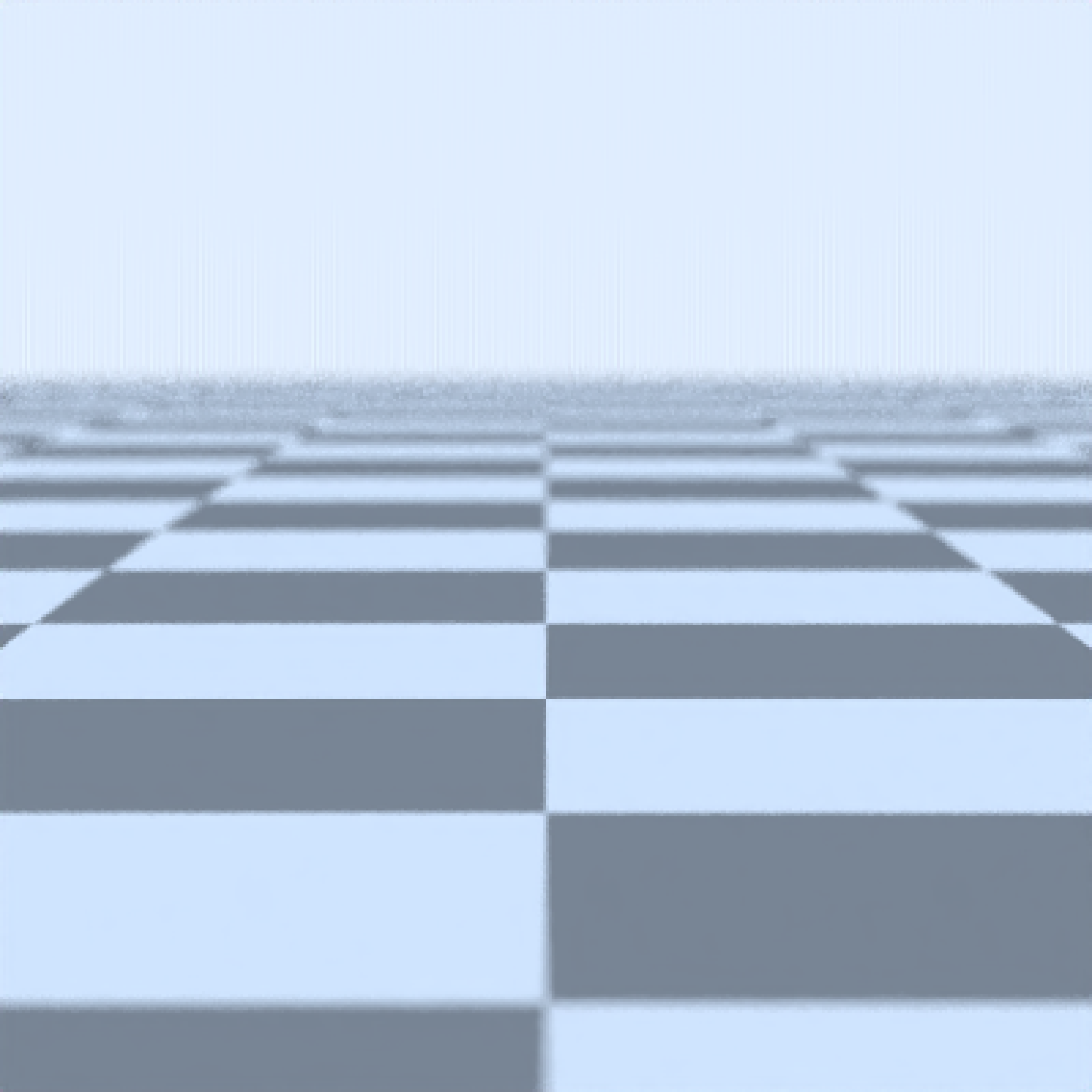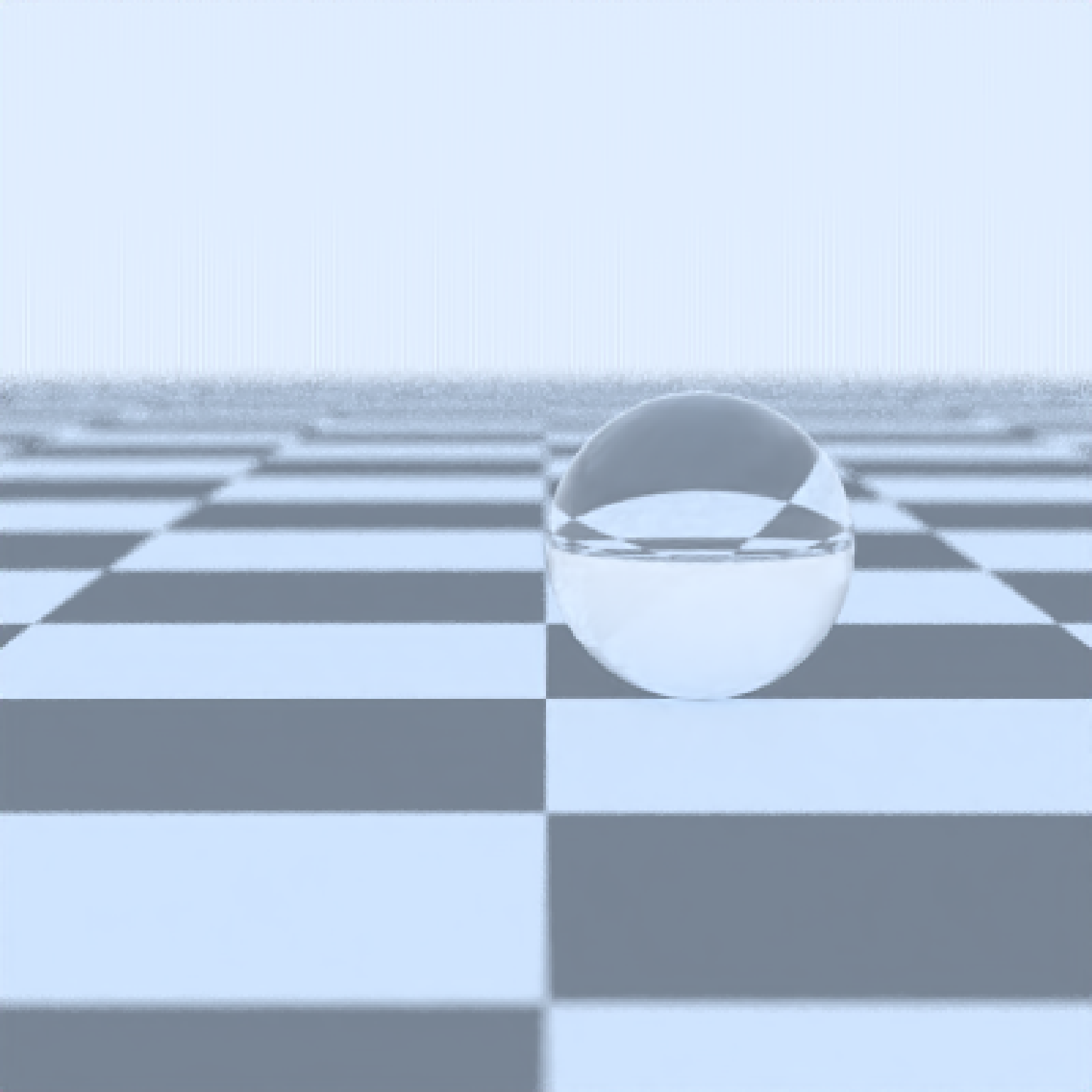Dielectric (glass) Material

dielectric(color = "white", refraction = 1.5,
implicit_sample = FALSE)

## Arguments

color Default white. The color of the surface. Can be either a hexadecimal code, R color string, or a numeric rgb vector listing three intensities between 0 and 1. Default 1.5. The index of refraction. Default TRUE. If FALSE, the object will not be sampled as part of the scattering probability density function.

## Value

Single row of a tibble describing the sphere in the scene.

## Examples

#Generate a checkered ground
scene = generate_ground(depth=-0.5,
material=lambertian(color="white", checkercolor="grey30",checkerperiod=2))render_scene(scene,parallel=TRUE)add_object(sphere(z=-0.5,radius=0.5,material=dielectric()))render_scene(scene,parallel=TRUE)add_object(cube(z=0.5,zwidth=0.5,material=dielectric(color="darkgreen"),angle=c(0,-45,0)))render_scene(scene,parallel=TRUE)material=lambertian(lightintensity=15),angle=c(0,45,0)))render_scene(scene,parallel=TRUE,aperture=0, ambient_light=FALSE,samples=1000)